# GMAT Math : DSQ: Calculating percents

## Example Questions

2 Next →

### Example Question #11 : Dsq: Calculating Percentsis 30% of, andis 30% ofis an integer. True or false:is an integer.

Statement 1:is an integer.

Statement 2:is divisible by 100.

BOTH statements TOGETHER are insufficient to answer the question.

Statement 1 ALONE is sufficient to answer the question, but Statement 2 ALONE is NOT sufficient to answer the question.

BOTH statements TOGETHER are sufficient to answer the question, but NEITHER statement ALONE is sufficient to answer the question.

Statement 2 ALONE is sufficient to answer the question, but Statement 1 ALONE is NOT sufficient to answer the question.

EITHER statement ALONE is sufficient to answer the question.

EITHER statement ALONE is sufficient to answer the question.

Explanation:

Assume Statement 1 alone.

Ifis 30% ofandis 30% of, then: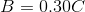and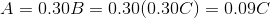or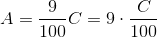is an integer; forto be an integer,must be divisible by 100. This makes Statement 2 a consequence of Statement 1, so we can now test Statement 2 alone.

Ifis divisible by 100, then for some integer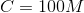. 30% of this is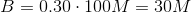is 30 times some integer, and is therefore an integer itself.

Either statement alone answers the question.

### Example Question #12 : Dsq: Calculating Percentsis 20% of, andis 20% ofis an integer. True or false:is an integer.

Statement 1:is not an integer.

Statement 2:is divisible by 72.

Statement 1 ALONE is sufficient to answer the question, but Statement 2 ALONE is NOT sufficient to answer the question.

Statement 2 ALONE is sufficient to answer the question, but Statement 1 ALONE is NOT sufficient to answer the question.

BOTH statements TOGETHER are sufficient to answer the question, but NEITHER statement ALONE is sufficient to answer the question.

BOTH statements TOGETHER are insufficient to answer the question.

EITHER statement ALONE is sufficient to answer the question.

BOTH statements TOGETHER are insufficient to answer the question.

Explanation:

Assume both statements are true.

Case 1: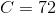Thenis 20% of this, so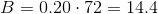is 20% of, so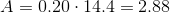Case 2: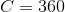Thenis 20% of this, so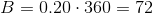is 20% of, so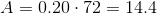In both situations, the conditions of the problem, as well as both statements, are true, but in one case,is not an integer and in the other case,is an integer. The statements together are inconclusive.

### Example Question #11 : Percentsis 25% of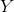, which is 25% of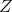is a positive integer.

True of false:is an integer.

Statement 1:is a prime number.

Statement 2:is an odd number.

Statement 2 ALONE is sufficient to answer the question, but Statement 1 ALONE is NOT sufficient to answer the question.

EITHER statement ALONE is sufficient to answer the question

BOTH statements TOGETHER are sufficient to answer the question, but NEITHER statement ALONE is sufficient to answer the question.

Statement 1 ALONE is sufficient to answer the question, but Statement 2 ALONE is NOT sufficient to answer the question.

BOTH statements TOGETHER are insufficient to answer the question.

EITHER statement ALONE is sufficient to answer the question

Explanation:is 25% of, so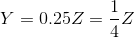.

Similarly,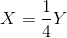.

Therefore,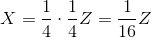,

or, equivalently,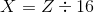or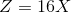.

Ifis an integer, then 16 is a factor of. This contradicts both Statement 1, since 16 is composite, makingcomposite, and Statement 2, since this makesa multiple of- that is, even. Either statement alone answers the question in the negative.

### Example Question #14 : Dsq: Calculating Percentsis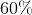of, andisof.

True or false: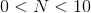.

Statement 1: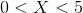.

Statement 2: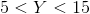EITHER statement ALONE is sufficient to answer the question.

Statement 1 ALONE is sufficient to answer the question, but Statement 2 ALONE is NOT sufficient to answer the question.

BOTH statements TOGETHER are sufficient to answer the question, but NEITHER statement ALONE is sufficient to answer the question.

Statement 2 ALONE is sufficient to answer the question, but Statement 1 ALONE is NOT sufficient to answer the question.

BOTH statements TOGETHER are insufficient to answer the question.

EITHER statement ALONE is sufficient to answer the question.

Explanation:is 60% of, meaning that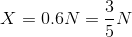, or, equivalently,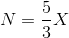.

From Statement 1 alone, since, it follows that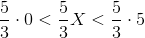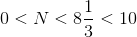This is enough to prove that.is 60% of, so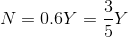.

From Statement 2 alone, since, it follows that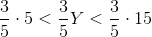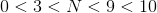.

This is enough to prove that.

### Example Question #15 : Dsq: Calculating Percents

Three candidates - Rodger, Stephanie, and Tina - ran for student body president. By the rules, the candidate who wins more than half the ballots cast wins the election outright; if no candidate wins more than half, there must be a runoff between the two top vote-getters. You may assume that no other names were written in.

Was there an outright winner, or will there be a runoff?

Statement 1: Rodger won 100 more votes than Stephanie and 210 more votes than Tina.

Statement 2: Tina won 25.9% of the votes.

STATEMENT 1 ALONE provides sufficient information to answer the question, but STATEMENT 2 ALONE does NOT provide sufficient information to answer the question.

BOTH STATEMENTS TOGETHER do NOT provide sufficient information to answer the question.

EITHER STATEMENT ALONE provides sufficient information to answer the question.

STATEMENT 2 ALONE provides sufficient information to answer the question, but STATEMENT 1 ALONE does NOT provide sufficient information to answer the question.

BOTH STATEMENTS TOGETHER provide sufficient information to answer the question, but NEITHER STATEMENT ALONE provides sufficient information to answer the question.

BOTH STATEMENTS TOGETHER provide sufficient information to answer the question, but NEITHER STATEMENT ALONE provides sufficient information to answer the question.

Explanation:

For one candidate to have won the election outright, (s)he must win more than 50% of the votes.

Statement 1 alone does not prove there was an outright winner. For example, if Rodger got 211 votes, then Stephanie got 111 votes, and Tina got 1 vote; this makes Rodger's share of the votes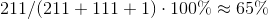,

making Rodger the outright winner. But if Rodger got 500 votes, then Stephanie got 400 votes, and Tina got 290; Rodger got the most votes, but his share is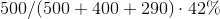,

not the required share of the vote.

Statement 2 alone does not prove this either, since 74.1% of the vote was won by either Rodger or Stephanie, but it is not specified how this is distributed; Roger or Stephanie could have won 51%, with the remaining 23.1% won by the other, resulting in an outright winner. However, it is also possible that each won half this, or about 37%, resulting in a runoff between the two.

Now assume both statements are true. Letbe the number of votes won by Tina. Then Rodger won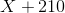votes and Stephanie won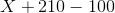, or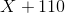, votes. The total votes are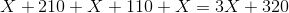.

Since Tina won 25.9% of the vote, we can set up an equation: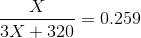This can be solved for. From this, the number of votes each candidate got, the votes cast, and, finally, the percent of the vote each won can be calculated.

2 Next →

### All GMAT Math Resources